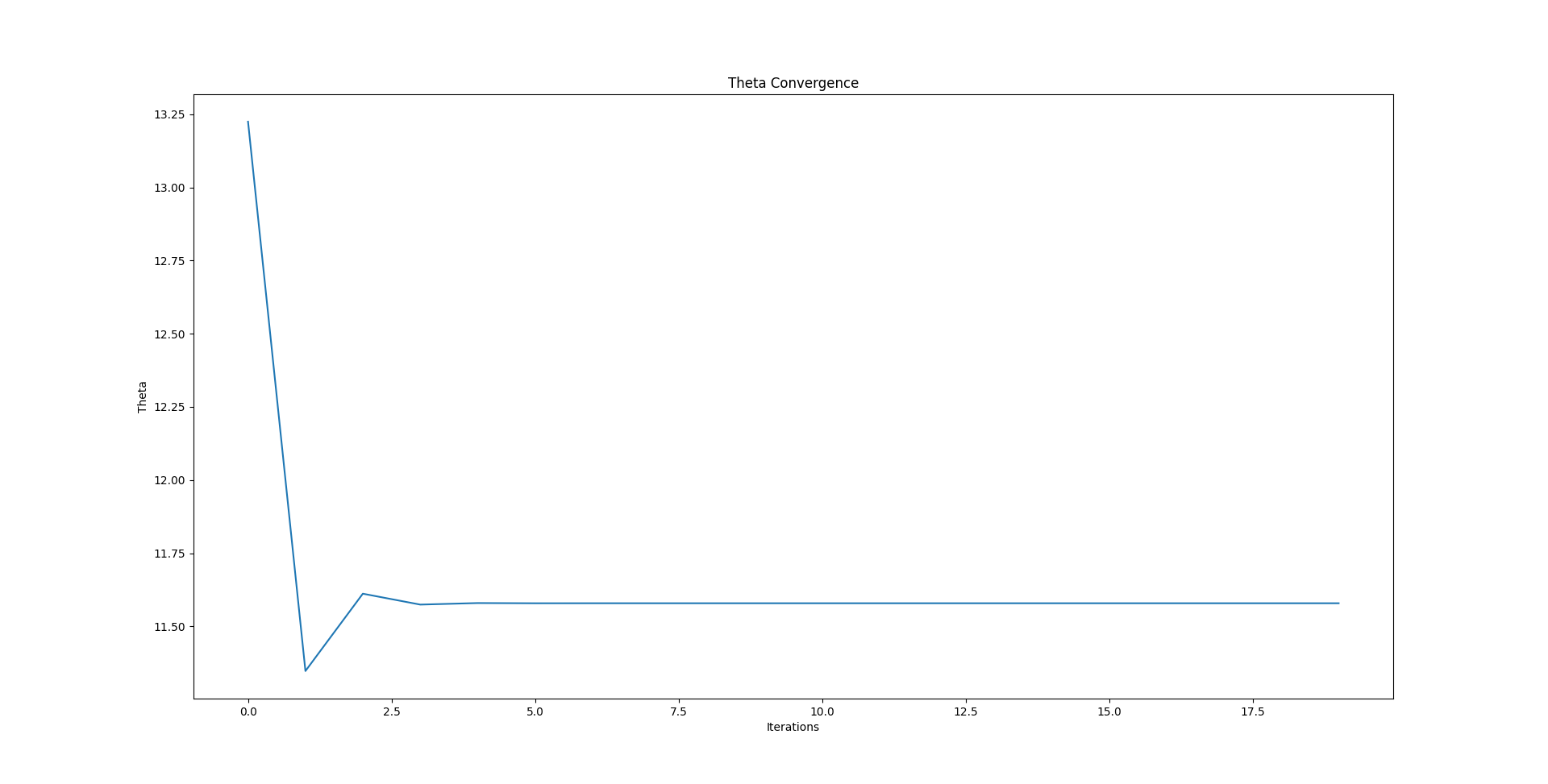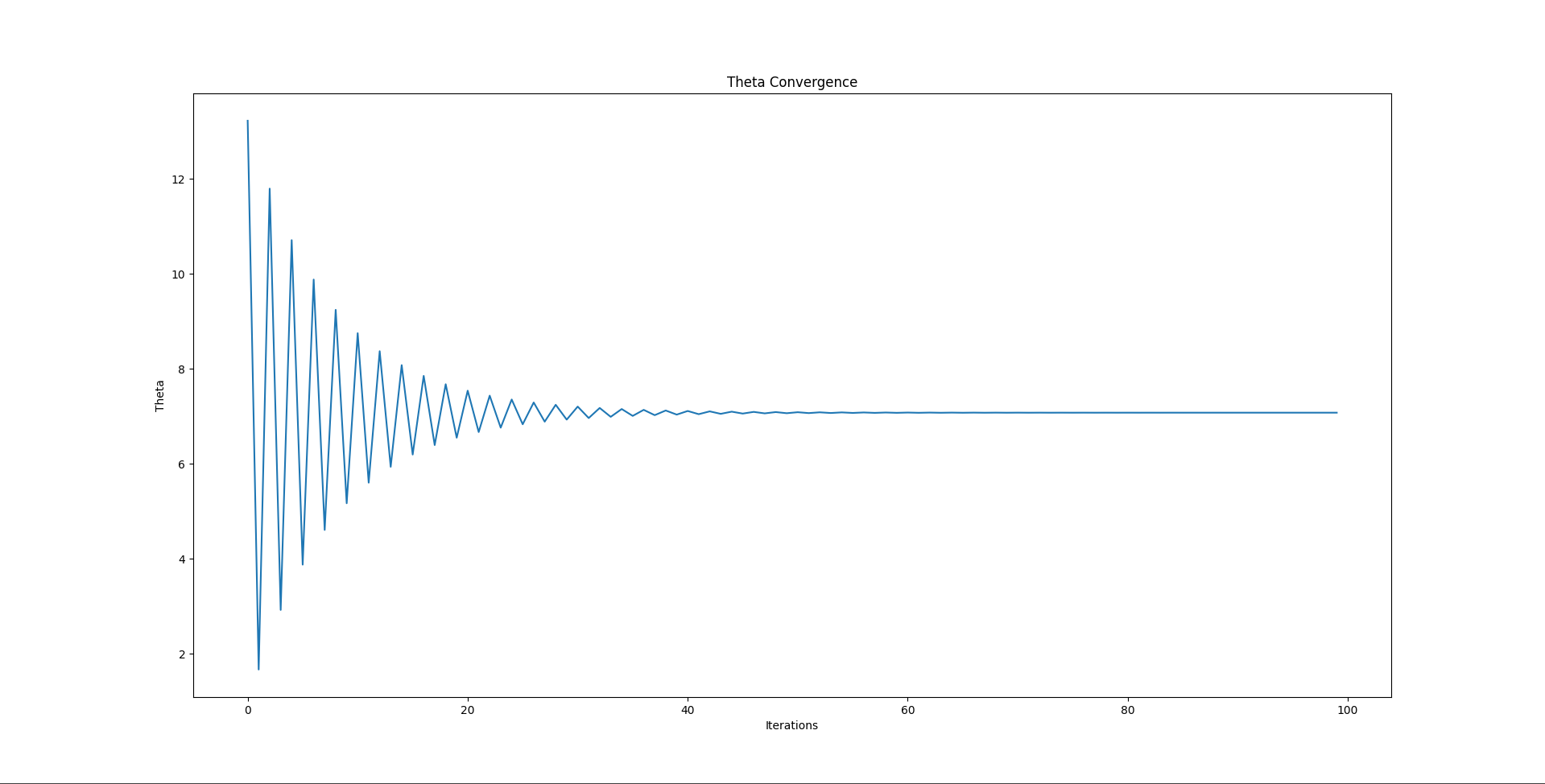# Accounting For Initial Height

Tags: think and control
Personhours: 2
Accounting For Initial Height By Mahesh

### Task: Account For Initial Height

In the previous trajectory calculations post, "Derive And Translate Trajectory Calculations Into Code", we did not take into account how the length of the launcher would effect our calculations. In reality, the height the disk would have to travel would be shortened by the launcher, since when titled at an angle the vertical distance would be shortened by $$lsin(\theta)$$, where $$l$$ represents the length of the launcher. This is because our launcher is mounted on a hinge, and therefore the rotation of the launcher affects the position where the disk leaves the launcher.

To take this into account, we would have to add $$lsin(\theta)$$ to the vertical distance the disk has to travel, however, $$\theta$$ depends on this distance as well. To solve this problem of circular dependency we can use an iterative method. This is because when $$\theta$$ is calculated for a given trajectory, the initial height of the disk will be raised by $$lsin(\theta)$$. This means the disk has to travel less distance vertically, meaning that $$\theta$$ will be smaller with the new launch height. But, when $$\theta$$ is decreased, so will the launch height, will in turn will raise theta, and so on. This process is convergent, meaning that iterating the process described above will eventually yield a $$\theta$$ whose change in the launch height is reflective of the launch height's change in theta. The following process can be used to find the convergent $$\theta$$:

1. Calculate $$v_0$$ and $$\theta$$ from the current launch height
2. Find the new launch height given the previously calculated $$\theta$$
3. Repeat steps 1-2

We graphed out the convergence of $$\theta$$ to confirm that these calculations would work correctly. Below is an image of the convergence of $$\theta$$ given a distance of 4 meters and a launcher length of 0.3 meters:To exaggerate the effect here is another image with a launcher length of 1.8 meters:### Next Steps:

The next step is to implement this in code to account for the height of the launcher, a fairly simple task. Tuning the iterations for performance vs accuracy won't really be necessary since $$\theta$$ seems to converge exponentially and iterations happen very quick on modern hardware anyway.

One concern still exists which is that the horizontal distance the disk has to travel is also effected by the launch angle $$\theta$$. However, this would only effect the distance by a matter of fractions of a centimeter, so it is likely not a big problem. If significant errors are encountered which cannot be traced down to other hardware or software defects/bugs then we can consider taking into account the effect that $$\theta$$ has on distance, and vice versa.

Date | February 6, 2021